# Language Identification using the ‘fastText’ package (a Benchmark)

#### 2022-04-07

In this blog post, I’ll explain how to perform Language Identification with the fastText R package and I’ll create a benchmark by including other language identification R packages, i.e.

• cld2, R Wrapper for Google’s Compact Language Detector 2
• cld3, Bindings to Google’s Compact Language Detector 3
• textcat, N-Gram Based Text Categorization
• franc, Port of the JavaScript ‘franc’, Detect the Language of Text

I didn’t use any packages that make calls to API’s because the dataset I’ll utilize consists of more than 50.000 thousand observations.

Based on my search on the web, currently, the WiLI benchmark dataset is among the ones that contain multilingual texts (Wikipedia extracts of 235 languages) and the corresponding labels as well. It can be downloaded either from Zenodo or from my Datasets Github repository.

We currently live in the Covid-19 Era and there are many human rights violation incidents (more often than before), therefore I decided to include in this benchmark also the human rights declarations of the 3 most spoken languages (Chinese, Enlish, Spanish) because they are more relevant than ever. The human rights declarations of the mentioned languages can be downloaded either from the official website or from my Datasets Github repository, and I think it deserves a read from time to time so that we don’t forget our rights as human beings.

### Benchmark based on the WiLI dataset

The fastText language identification pre-trained models support currently 176 languages. The following character vector shows the available language isocodes.

``````fasttext_supported_languages = c('af', 'als', 'am', 'an', 'ar', 'arz', 'as', 'ast', 'av',
'az', 'azb', 'ba', 'bar', 'bcl', 'be', 'bg', 'bh', 'bn',
'bo', 'bpy', 'br', 'bs', 'bxr', 'ca', 'cbk', 'ce', 'ceb',
'ckb', 'co', 'cs', 'cv', 'cy', 'da', 'de', 'diq', 'dsb',
'dty', 'dv', 'el', 'eml', 'en', 'eo', 'es', 'et', 'eu',
'fa', 'fi', 'fr', 'frr', 'fy', 'ga', 'gd', 'gl', 'gn',
'gom', 'gu', 'gv', 'he', 'hi', 'hif', 'hr', 'hsb', 'ht',
'hu', 'hy', 'ia', 'id', 'ie', 'ilo', 'io', 'is', 'it',
'ja', 'jbo', 'jv', 'ka', 'kk', 'km', 'kn', 'ko', 'krc',
'ku', 'kv', 'kw', 'ky', 'la', 'lb', 'lez', 'li', 'lmo',
'lo', 'lrc', 'lt', 'lv', 'mai', 'mg', 'mhr', 'min', 'mk',
'ml', 'mn', 'mr', 'mrj', 'ms', 'mt', 'mwl', 'my', 'myv',
'mzn', 'nah', 'nap', 'nds', 'ne', 'new', 'nl', 'nn', 'no',
'oc', 'or', 'os', 'pa', 'pam', 'pfl', 'pl', 'pms', 'pnb',
'ps', 'pt', 'qu', 'rm', 'ro', 'ru', 'rue', 'sa', 'sah',
'sc', 'scn', 'sco', 'sd', 'sh', 'si', 'sk', 'sl', 'so',
'sq', 'sr', 'su', 'sv', 'sw', 'ta', 'te', 'tg', 'th', 'tk',
'tl', 'tr', 'tt', 'tyv', 'ug', 'uk', 'ur', 'uz', 'vec',
'vep', 'vi', 'vls', 'vo', 'wa', 'war', 'wuu', 'xal', 'xmf',
'yi', 'yo', 'yue', 'zh')``````

For illustration purposes we’ll subset the WiLI dataset to the 2-letter isocodes of the supported fastText languages. For this purpose we’ll use the ISOcodes R package and especially the ISO_639_2 function which includes the required 2- and 3-letter isocodes and also the available full names of the languages,

``````isocodes = ISOcodes::ISO_639_2

comp_cases = complete.cases(isocodes\$Alpha_2)
isocodes_fasttext = isocodes[comp_cases, ]
# dim(isocodes_fasttext)

idx_keep_fasttext = which(isocodes_fasttext\$Alpha_2 %in% fasttext_supported_languages)

isocodes_fasttext = isocodes_fasttext[idx_keep_fasttext, ]
isocodes_fasttext = data.table::data.table(isocodes_fasttext)
# isocodes_fasttext

lower_nams = tolower(isocodes_fasttext\$Name)
lower_nams = trimws(as.vector(unlist(lapply(strsplit(lower_nams, "[;, ]"), function(x) x))), which = 'both')   # remove second or third naming of the country name

isocodes_fasttext\$Name_tolower = lower_nams
isocodes_fasttext``````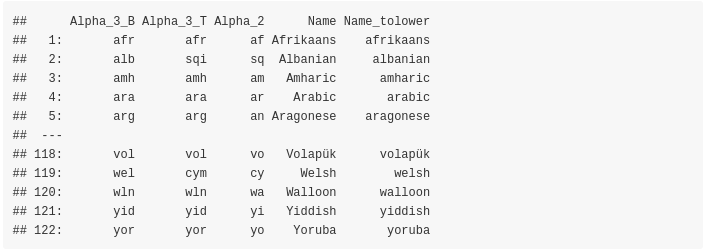The next function will be used to compute and print the accuracy in all cases,

``````print_accuracy = function(size_input_data,
true_data,
preds_data,
method) {

cat(glue::glue("Total Rows: {size_input_data}"), '\n')
rnd_2 = round(length(preds_data)/size_input_data, 4)
msg_2 ="Predicted Rows: {length(preds_data)}  ({rnd_2 * 100}% predicted)"
cat(glue::glue(msg_2), '\n')
cat(glue::glue("Missing Values:  {size_input_data - length(preds_data)}"), '\n')
rnd_4 = round(sum(true_data == preds_data) / length(preds_data), 4)
msg_4 = "Accuracy on 'Predicted Rows' using '{method}':  {rnd_4 * 100}%"
cat(glue::glue(msg_4), '\n')
}

# fasttext language identification supported languages as described in https://fasttext.cc/docs/en/language-identification.html``````

As mentioned earlier the WiLI benchmark dataset can be downloaded either from Zenodo or from my Datasets Github repository. Once downloaded and unzipped the folder includes the following files (for the remaining of this blog post I’ll assume that the dir_wili_2018 variable points to the WiLI data directory ),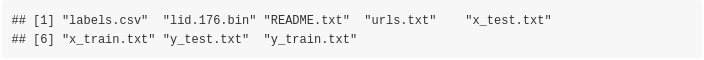For this benchmark we’ll use only the test data (‘x_test.txt’ and ‘y_test.txt’ files) and we’ll keep only the WiLI-isocodes that intersect with the fastText isocodes,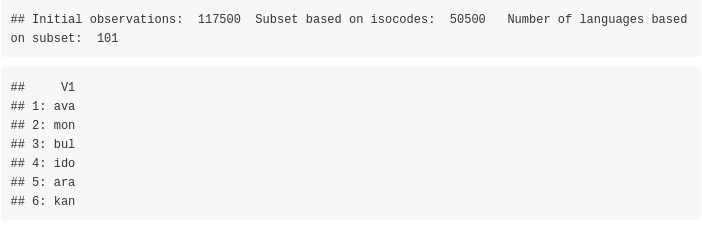### fastText based on the smaller pre-trained model ‘lid.176.ftz’ (approx. 917 kB)

First, we’ll use the smaller pre-trained dataset,

``````file_ftz = system.file("language_identification/lid.176.ftz", package = "fastText")

dtbl_res_in = fastText::language_identification(input_obj = wili_test_x\$V1,
pre_trained_language_model_path = file_ftz,
k = 1,
th = 0.0,
verbose = TRUE)``````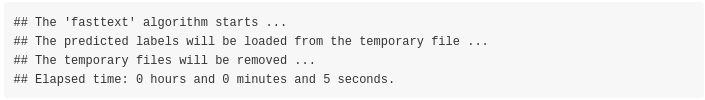``````dtbl_res_in\$true_label = wili_test_y\$V1
# dtbl_res_in

isocodes_fasttext_subs = isocodes_fasttext[, c(1,3)]   # merge the predicted labels with the 3-letter isocodes

merg_labels = merge(dtbl_res_in, isocodes_fasttext_subs, by.x = 'iso_lang_1', by.y = 'Alpha_2')
# as.vector(colSums(is.na(merg_labels)))

print_accuracy(size_input_data = nrow(wili_test_y),
true_data = merg_labels\$true_label,
preds_data = merg_labels\$Alpha_3_B,
method = 'fastText (.ftz pre-trained model)')``````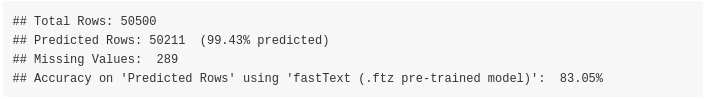The accuracy of the model was 83.05% (on 50211 out of 50500 text extracts)

### fastText based on the bigger pre-trained model ‘lid.176.bin’ (approx. 126 MB)

Let’s move to the bigger pre-trained model which is mentioned to be more accurate. This model can be downloaded either from the official website or from my Datasets Github repository. The parameter setting of the fastText::language_identification() function is the same as before, and the only thing that changes is the pre_trained_language_model_path parameter which is set to lid.176.bin. Assuming this file is downloaded and extracted in the dir_wili_2018 directory then,

``````file_bin = file.path(dir_wili_2018, 'lid.176.bin')

dtbl_res_in = fastText::language_identification(input_obj = wili_test_x\$V1,
pre_trained_language_model_path = file_bin,
k = 1,
th = 0.0,
verbose = TRUE)``````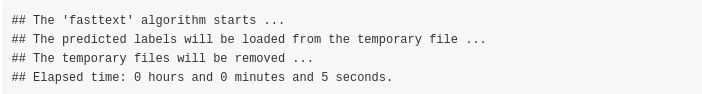``````dtbl_res_in\$true_label = wili_test_y\$V1
# dtbl_res_in

isocodes_fasttext_subs = isocodes_fasttext[, c(1,3)]   # merge the predicted labels with the 3-letter isocodes

merg_labels = merge(dtbl_res_in, isocodes_fasttext_subs, by.x = 'iso_lang_1', by.y = 'Alpha_2')
# as.vector(colSums(is.na(merg_labels)))

print_accuracy(size_input_data = nrow(wili_test_y),
true_data = merg_labels\$true_label,
preds_data = merg_labels\$Alpha_3_B,
method = 'fastText (.ftz pre-trained model)')``````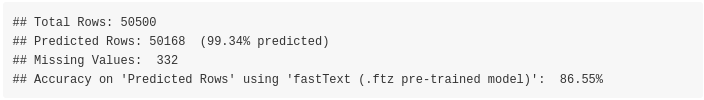The accuracy based on the bigger model was increased to 86.55% (on 50168 out of 50500 text extracts)

### ggplot visualization of the bigger .bin model

The following plot shows the confusion matrix of the bigger .bin model. The main diagonal is dominated by the dark green color indicating higher accuracy rates,

``````tbl = table(merg_labels\$true_label, merg_labels\$Alpha_3_B)

df = as.data.frame.table(tbl)
colnames(df) = c('country_vert', 'country_horiz', 'Freq')

require(magrittr)
require(dplyr)
require(ggplot2)

df <- df %>%
mutate(country_vert = factor(country_vert),                 # alphabetical order by default
country_horiz = factor(country_horiz, levels = rev(unique(country_horiz))))

plt_tbl = ggplot(df, aes(x=country_vert, y=country_horiz, fill=Freq)) +
geom_tile() + theme_bw() + coord_equal() +
scale_fill_distiller(palette="Greens", direction=1) +
ggplot2::theme(axis.text.x = element_text(angle = 45, vjust = 1.0, hjust = 1.0))

plt_tbl``````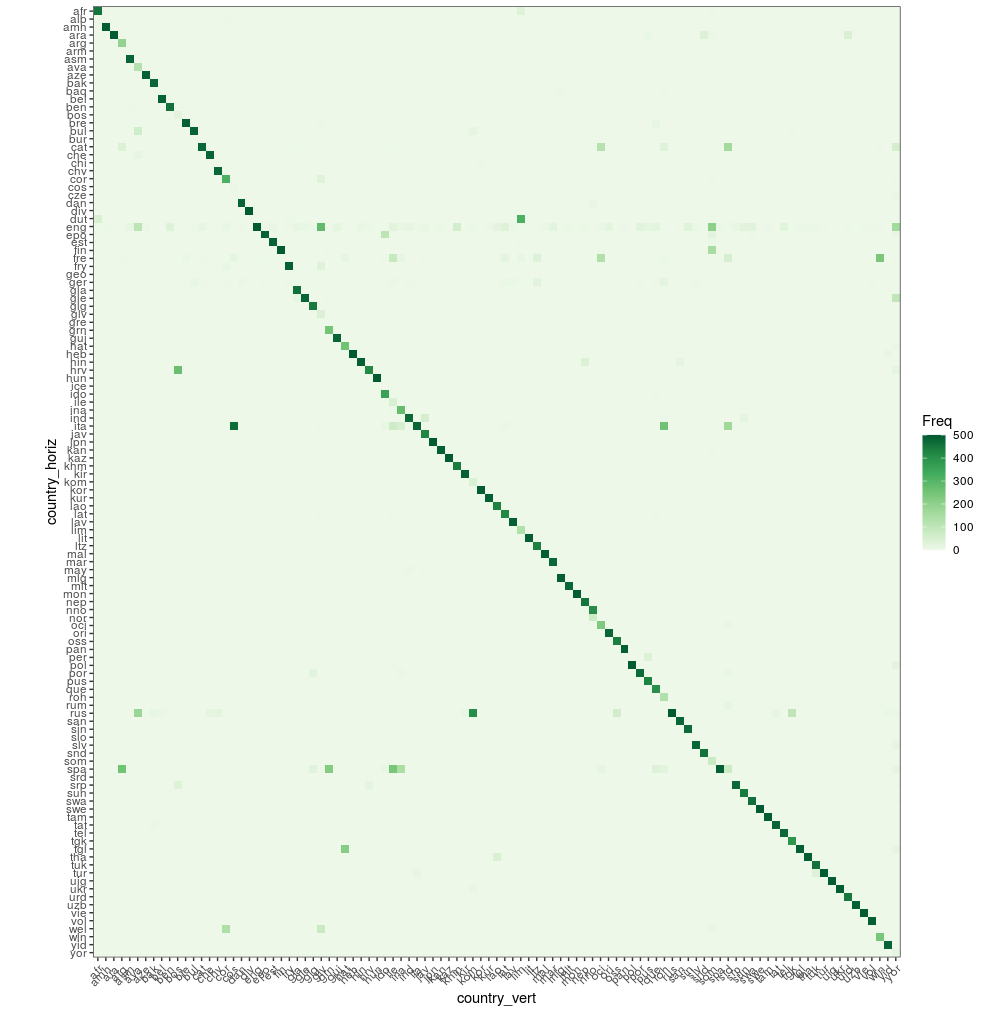### ‘cld2’ language recognition package

The Google’s Compact Language Detector 2 (CLD2) " … probabilistically detects over 80 languages in Unicode UTF-8 text, either plain text or HTML/XML. For mixed-language input, CLD2 returns the top three languages found and their approximate percentages of the total text bytes (e.g. 80% English and 20% French out of 1000 bytes) …“. Based on the R package documentation,”The function ‘detect_language()’ is vectorised and guesses the language of each string in text or returns NA if the language could not reliably be determined."

``````require(cld2)

t_start = proc.time()
cld2_vec = cld2::detect_language(text = wili_test_x\$V1, plain_text = TRUE, lang_code = TRUE)

cld2_dtbl = data.table::setDT(list(Alpha_2 = cld2_vec))
cld2_dtbl\$true_label = wili_test_y\$V1

merg_labels_cld2 = merge(cld2_dtbl, isocodes_fasttext_subs, by = 'Alpha_2')
# as.vector(colSums(is.na(merg_labels_cld2)))

print_accuracy(size_input_data = nrow(wili_test_y),
true_data = merg_labels_cld2\$true_label,
preds_data = merg_labels_cld2\$Alpha_3_B,
method = 'cld2')``````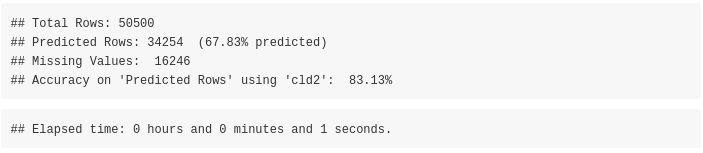The accuracy of the cld2 package is 83.13% (on 34254 out of 50500 text extracts)

### ‘cld3’ language recognition package

The " … Google’s Compact Language Detector 3 is a neural network model for language identification and the successor of CLD2 (available from) CRAN. This version is still experimental and uses a novell algorithm with different properties and outcomes. For more information see: https://github.com/google/cld3#readme …“. Based on the R package documentation,”The function detect_language() is vectorised and guesses the language of each string in text or returns NA if the language could not reliably be determined."

``````require(cld3)

t_start = proc.time()
cld3_vec = cld3::detect_language(text = wili_test_x\$V1)

cld3_dtbl = data.table::setDT(list(Alpha_2 = cld3_vec))
cld3_dtbl\$true_label = wili_test_y\$V1

merg_labels_cld3 = merge(cld3_dtbl, isocodes_fasttext_subs, by = 'Alpha_2')
# as.vector(colSums(is.na(merg_labels_cld3)))

print_accuracy(size_input_data = nrow(wili_test_y),
true_data = merg_labels_cld3\$true_label,
preds_data = merg_labels_cld3\$Alpha_3_B,
method = 'cld3')``````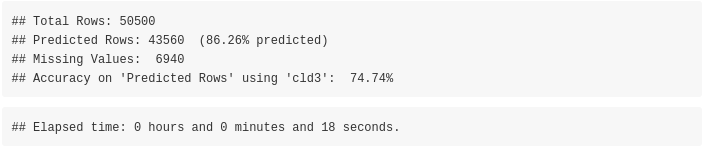The accuracy of the cld3 package is 74.74% (on 43560 out of 50500 text extracts)

### Language recognition using the ‘textcat’ R package

The ‘textcat’ R package performs ‘text categorization based on n-grams’. The documentation of the package mentions: "… TextCat (https://www.let.rug.nl/vannoord/TextCat/) is a Perl implementation of the Cavnarand Trenkle ‘N-Gram-Based Text Categorization’ technique by Gertjan van Noord which was subsequently integrated into SpamAssassin. It provides byte n-gram profiles for 74 ‘languages’ (more precisely, language/encoding combinations). The C library reimplementation libtextcat (https://software.wise-guys.nl/libtextcat/) adds one more non-empty profile.

• ‘TC_byte_profiles’ provides these byte profiles.
• ‘TC_char_profiles’ provides a subset of 56 character profiles obtained by converting the byte sequences to UTF-8 strings where possible.

The category ids are unchanged from the original, and give the full (English) name of the language, optionally combined the name of the encoding script. Note that ‘scots’ indicates Scots, the Germanic language variety historically spoken in Lowland Scotland and parts of Ulster, to be distinguished from Scottish Gaelic (named ‘scots_gaelic’ in the profiles), the Celtic language variety spoken in most of the western Highlands and in the Hebrides (see https://en.wikipedia.org/wiki/Scots_language) …"

Apart from the previous 2 mentioned TC-profiles the ECIMCI_profiles (26 profiles) also exists. In my benchmark I’ll use only the ‘TC_byte_profile’ (75 profiles) and ‘TC_char_profiles’ (56 profiles) as input to the textcat() function to compute the country names, which by default the textcat() function returns. I’ll wrap the function to parallel::mclapply() because I observed it returns the results faster using multiple threads (in my benchmark I used 8 threads).

#### using the ‘TC_byte_profiles’

Before proceeding lets have a look to the available profiles,

``````threads = parallel::detectCores()
require(textcat)

names(textcat::TC_byte_profiles)``````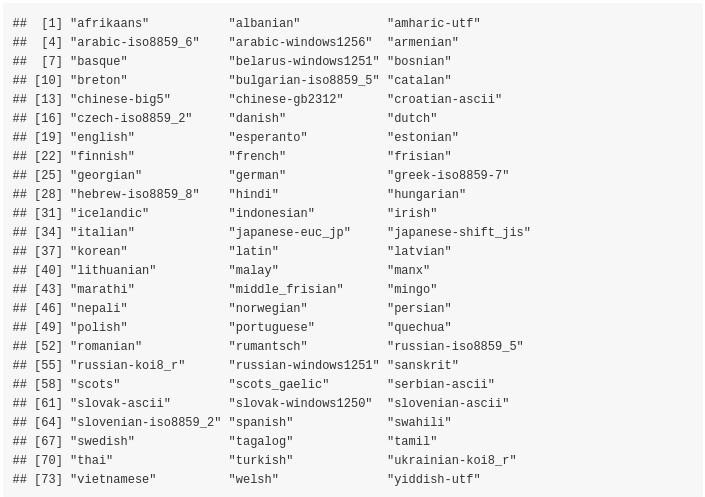What we want is that the initial lowercase isocodes intersect with the processed TC_byte_profiles so that the computation of the accuracy is correct,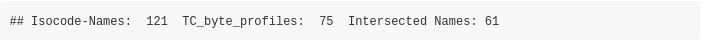``````t_start = proc.time()
textc = as.vector(unlist(parallel::mclapply(1:length(wili_test_x\$V1), function(x) {
textcat(x = wili_test_x\$V1[x], p = textcat::TC_byte_profiles, method = "CT")

textc = as.vector(unlist(lapply(strsplit(textc, '-'), function(x) x)))
textc = trimws(textc, which = 'both')

unique(textc)``````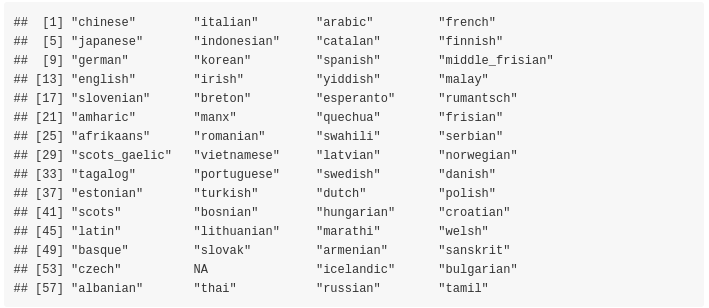``````textc_dtbl = data.table::setDT(list(Name_tolower = textc))
textc_dtbl\$true_label = wili_test_y\$V1

fasttext_isoc_name = isocodes_fasttext[, c(1,5)]
merg_labels_textc = merge(textc_dtbl, fasttext_isoc_name, by = 'Name_tolower')
# as.vector(colSums(is.na(merg_labels_cld2)))

print_accuracy(size_input_data = nrow(wili_test_y),
true_data = merg_labels_textc\$true_label,
preds_data = merg_labels_textc\$Alpha_3_B,
method = 'textcat ( TC_byte_profiles )')``````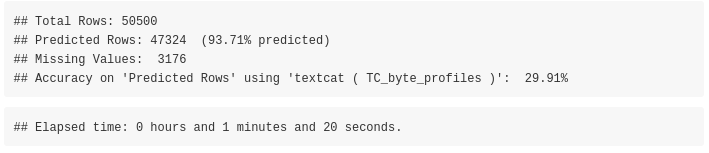The accuracy of the textcat package using the TC_byte_profiles is 29.91% (on 47324 out of 50500 text extracts)

#### using the ‘TC_char_profiles’

Again, as previously we can have a look to the available profiles,

``names(textcat::TC_char_profiles)``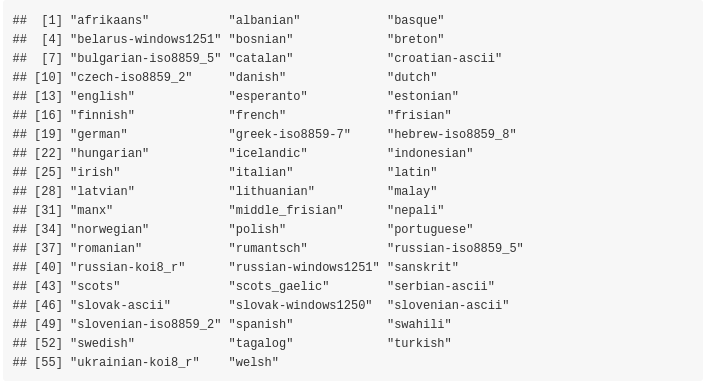What we want is that the initial lowercase isocodes intersect with the processed TC_char_profiles so that the computation of the accuracy is correct,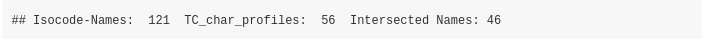``````t_start = proc.time()
textc = as.vector(unlist(parallel::mclapply(1:length(wili_test_x\$V1), function(x) {
textcat(x = wili_test_x\$V1[x], p = textcat::TC_char_profiles, method = "CT")

textc = as.vector(unlist(lapply(strsplit(textc, '-'), function(x) x)))
textc = trimws(textc, which = 'both')

unique(textc)``````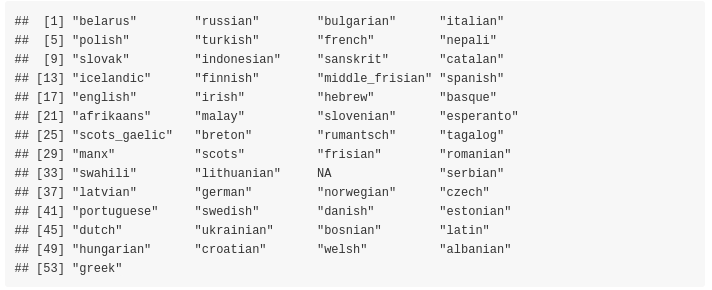``````textc_dtbl = data.table::setDT(list(Name_tolower = textc))
textc_dtbl\$true_label = wili_test_y\$V1

fasttext_isoc_name = isocodes_fasttext[, c(1,5)]
merg_labels_textc = merge(textc_dtbl, fasttext_isoc_name, by = 'Name_tolower')
# as.vector(colSums(is.na(merg_labels_cld2)))

print_accuracy(size_input_data = nrow(wili_test_y),
true_data = merg_labels_textc\$true_label,
preds_data = merg_labels_textc\$Alpha_3_B,
method = 'textcat ( TC_char_profiles )')``````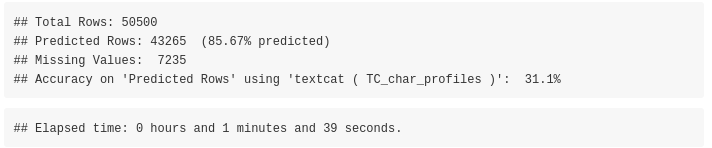The accuracy of the textcat package using the TC_char_profiles is 31.10% (on 43265 out of 50500 text extracts)

### Language recognition using the ‘franc’ R package

The R package port of Franc has no external dependencies and supports 310 languages. All languages spoken by more than one million speakers. Franc is a port of the JavaScript project of the same name. Based on the documentation of the JavaScript project, " … franc supports many languages, which means it’s easily confused on small samples. Make sure to pass it big documents to get reliable results …"

The franc() function expects a text extract, therefore we will wrap the function with parallel::mclapply() as we’ve done with the textcat package to reduce the computation time. Moreover, we’ll set the min_speakers parameter to 0 to include all languages known by franc (increasing the max_length parameter to 4096 does not improve the accuracy for this specific data / text extracts),

``````require(franc)

t_start = proc.time()
franc_res = as.vector(unlist(parallel::mclapply(1:length(wili_test_x\$V1), function(x) {
franc(text = wili_test_x\$V1[x], min_speakers = 0, min_length = 10, max_length = 2048)

franc_dtbl = data.table::setDT(list(franc = franc_res, true_label = wili_test_y\$V1))
# as.vector(colSums(is.na(franc_dtbl)))

print_accuracy(size_input_data = nrow(wili_test_y),
true_data = franc_dtbl\$true_label,
preds_data = franc_dtbl\$franc,
method = 'franc')``````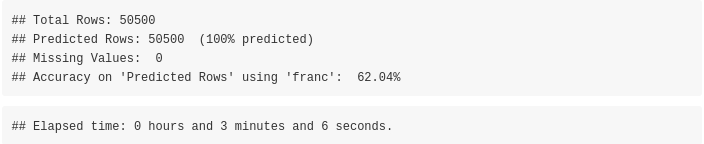The accuracy of the franc package is 62.04% (on 50500 out of 50500 text extracts)

### Overview datatable of all methods

Sorted by Accuracy (highest better),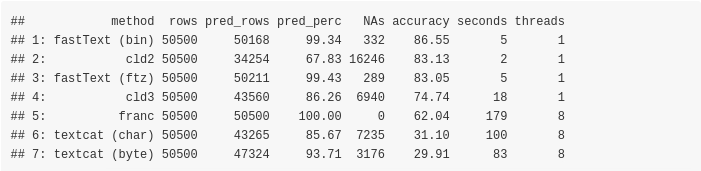Sorted by predicted percentage of text extracts (highest better),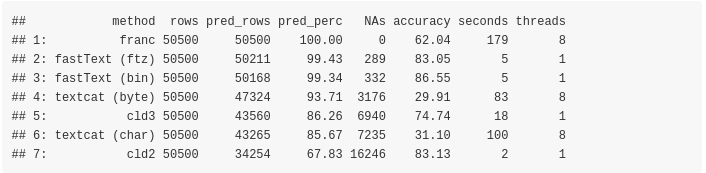Sorted by missing values (lowest better),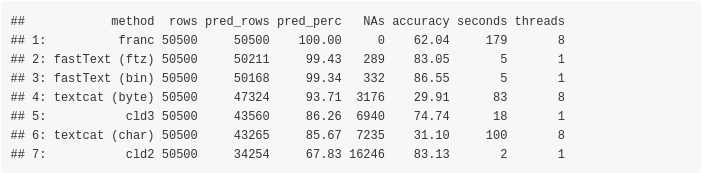Sorted by computation time (lowest better),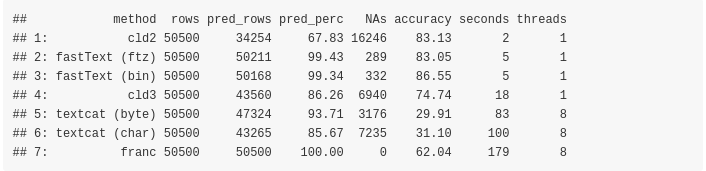### Benchmark based on the Human Rights Declaration files

We can test the mentioned functions also using the Declaration of Human Rights text files, which are smaller in size and can give hints on potential misclassifications. As I mentioned at the beginning of this blog post the data can be downloaded from two different internet sources. I’ll use only 3 files from the official website based on the total number of speakers worldwide (the first 3 are: Chinese, English, Spanish) and you can see the full list of the most spoken languages worldwide in the correponding wikipedia article.

Assuming the .zip file is downloaded and extracted in the dir_wili_2018 directory and the folder name that includes the files is named as declaration_human_rights then,

``dir_files = file.path(dir_wili_2018, 'declaration_human_rights')``
``````lst_files = list.files(dir_files, full.names = T, pattern = '.pdf')

decl_dat = lapply(1:length(lst_files), function(x) {

iter_dat = pdftools::pdf_text(pdf = lst_files[x])
lang = trimws(unlist(strsplit(gsub('.pdf', '', basename(lst_files[x])), '_')), which = 'both')
lang = lang[length(lang)]
vec_txt = as.vector(unlist(trimws(iter_dat, which = 'both')))
vec_txt = as.vector(sapply(vec_txt, function(x) gsub('\n', '', x)))

idx_lang = which(isocodes_fasttext\$Name_tolower == lang)
isocode_3_language = rep(isocodes_fasttext\$Alpha_3_B[idx_lang], length(vec_txt))
isocode_2_language = rep(isocodes_fasttext\$Alpha_2[idx_lang], length(vec_txt))
language = rep(lang, length(vec_txt))

dtbl = data.table::setDT(list(isocode_3_language = isocode_3_language,
isocode_2_language = isocode_2_language,
language = language,
text = vec_txt))
dtbl
})

decl_dat = data.table::rbindlist(decl_dat)``````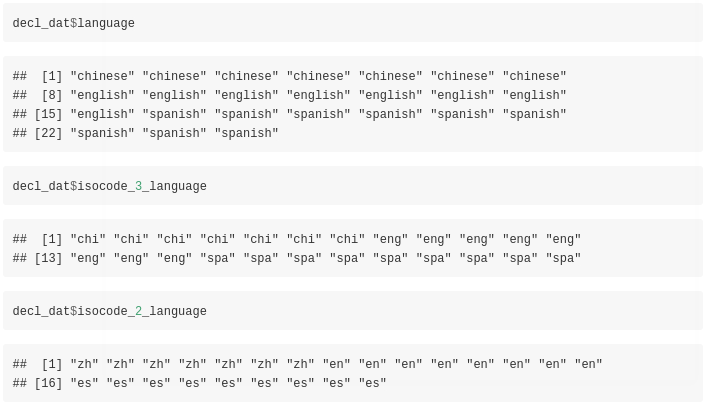The output data.table includes besides the language also the language isocodes (consisting of 2 and 3 letters) and the text extracts. We can start to identify the language of these extracts using the fastText R package and utilizing the small pre-trained ‘lid.176.ftz’ model,

``````dtbl_res_in = fastText::language_identification(input_obj = decl_dat\$text,
pre_trained_language_model_path = file_ftz,
k = 1,
th = 0.0,